A post from data.visualisation.free.fr

``````library(ggplot2)
library(ggthemes)
#library(doBy)
library(lubridate)
library(dplyr)
library(xtable)
library(reshape2)
###### --- General ----
Myroot <- "c:/Chris/Cours2016/MOOC/"
#Myroot <- "F:/ZChris/Cours2016/MOOC/"``````

### Why ``cheating’’ is sometimes useful in data science

Recently, I’ve worked on data from a MOOC we have created with some colleagues. The dataset was quite impressive since more than 3000 learners joined the course, viewed or interacted with some ressources (called ``steps‘’), posted comments and pass some tests. One of our goal was to create a data visualisation that alowed us to see the results of the learners’ tests, and, if possible, to detect some pattern in learners’ results over the 5 tests. The data set looks like that:

``sample_n(select(Scorebylearners, learner_id, step, test_score), 10)``

Using that dataset, we wanted to answer some questions:

Are there some visible patterns? Are learners with good results for one test still good at another?

So my first reflex was a plot with all the learners’results over the 5 steps:

``````Plot.Point <- ggplot(Scorebylearners, aes(x=step, y= test_score)) +
geom_point(color = "grey", alpha=0.80) +
scale_x_discrete(name="Test step number", limits=c("1.15", "2.12", "3.21" , "4.4.", "4.10")) +
scale_y_discrete(name ="Score",  limits=c(0,3,6,9, 12)) +
labs(title = "Learners' score for each test ",
subtitle = paste("N=", nrow(Scorebylearners), "learners - ", nrow(TestAnalysis),"observations"),
caption = "Source: MOOC ``Manage your prices'', FutureLearn (2017)"
) +
coord_cartesian(ylim = c(0,12)) +
theme_tufte()
Plot.Point``````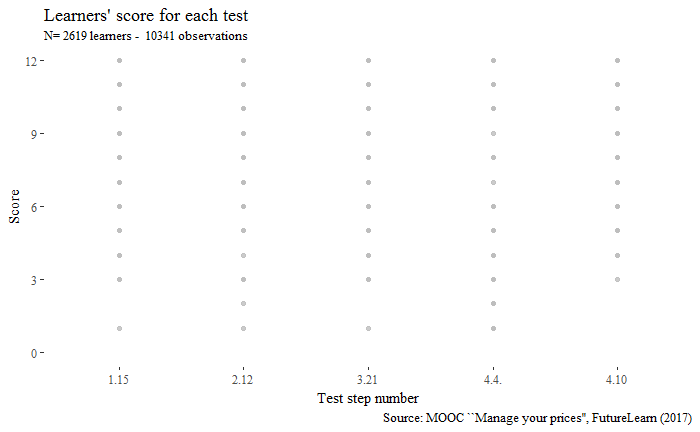Of course, the results to these tests are integers and take only some fixed values from 0 to 12 and many observations are overlapping.

This is a begginers’ mistake!

Well, so my second reflex was to use classical statistical representation such as the good old box-and-whiskers plot (boxplots)! .

``````Plot.Box <-  ggplot(Scorebylearners, aes(x=step, y= test_score)) +
geom_boxplot(outlier.colour= "grey", color= "darkgrey", fill="grey") +
guides(colour=FALSE, fill=FALSE)+
scale_x_discrete(name="Test step number", limits=c("1.15", "2.12", "3.21" , "4.4.", "4.10")) +
scale_y_discrete(name ="Score",  limits=c(0,3,6,9, 12)) +
labs(title = "Distribution of learners' score for each test (Box plot)",
subtitle = paste("N=", nrow(Scorebylearners), "learners - ", nrow(TestAnalysis),"observations"),
caption = "Source: MOOC ``Manage your prices'', FutureLearn (2017)"
) +
coord_cartesian(ylim = c(0,12)) +
theme_tufte()
Plot.Box``````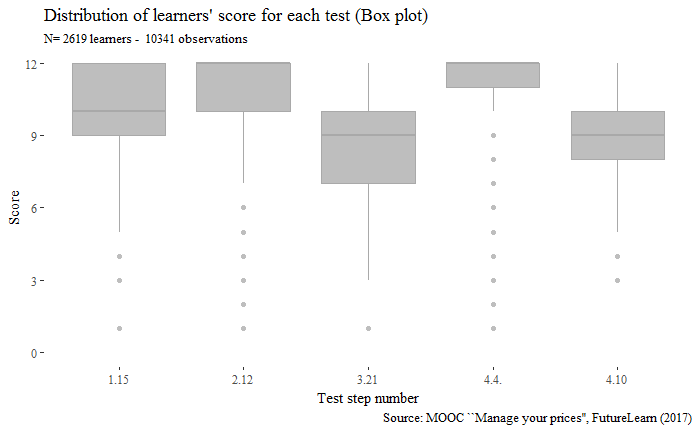That’s better, and I can see that there is some noticeable difference in the test results. But I wanted to see the individuals performances inside the boxes.

For that I have no choice but to cheat a little bit …

### Cheating a little bit by adding some random noise…

In order to avoid overlapping, there are 2 basic tricks: * to use transparency (or brushing, or alpha-transparency) * to jitter the data by adding some random component to either the horizontal or vertical component.

Let us add transparency and horizontal jitter only.

``````Plot.Jitter.H <- ggplot(Scorebylearners, aes(x=step, y= test_score)) +
geom_jitter(color = "grey", alpha=0.20, width=0.20, height = 0) +
scale_x_discrete(name="Test step number", limits=c("1.15", "2.12", "3.21" , "4.4.", "4.10")) +
scale_y_discrete(name ="Score",  limits=c(0,3,6,9, 12)) +
labs(title = "Learners' score for each test (horizontal jitter)",
subtitle = paste("N=", nrow(Scorebylearners), "learners - ", nrow(TestAnalysis),"observations"),
caption = "Source: MOOC ``Manage your prices'', FutureLearn (2017)"
) +
coord_cartesian(ylim = c(0,12)) +
theme_tufte()
Plot.Jitter.H``````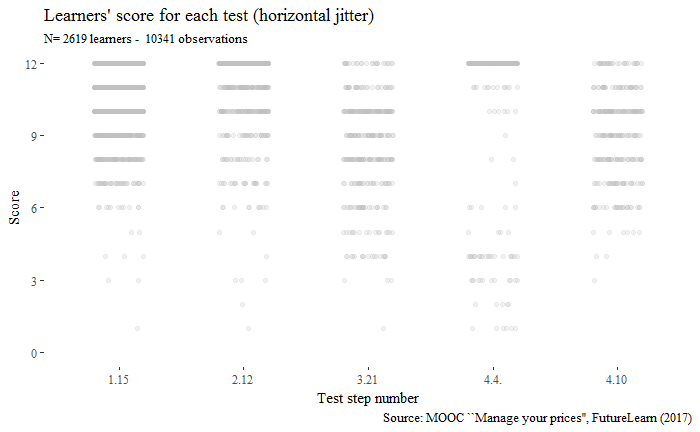The points we see now (thanks to jiter) are not the original ones. Is that cheating?

Let us do transparency and vertical jitter.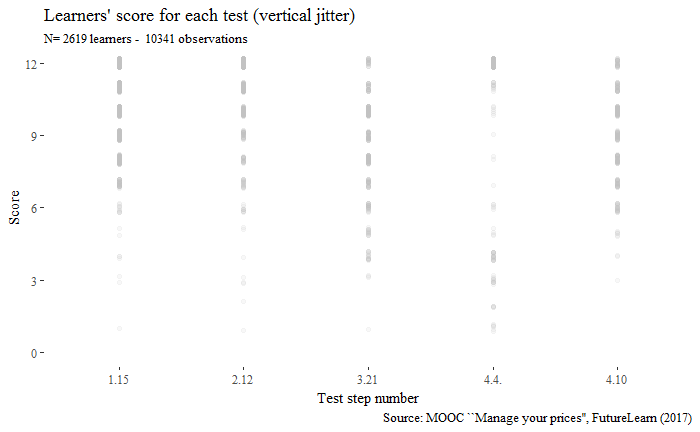Let us add transparency with horizontal and vertical jitter.

``````Plot.Jitter <- ggplot(Scorebylearners, aes(x=step, y= test_score)) +
geom_jitter(color = "grey", alpha=0.60, width=0.40) +
scale_x_discrete(name="Test step number", limits=c("1.15", "2.12", "3.21" , "4.4.", "4.10")) +
scale_y_discrete(name ="Score",  limits=c(0,3,6,9, 12)) +
labs(title = "Learners' score for each test (Horizontal + vertical jitter)",
subtitle = paste("N=", nrow(Scorebylearners), "learners - ", nrow(TestAnalysis),"observations"),
caption = "Source: MOOC ``Manage your prices'', FutureLearn (2017)"
) +
coord_cartesian(ylim = c(0,12)) +
theme_tufte()
Plot.Jitter``````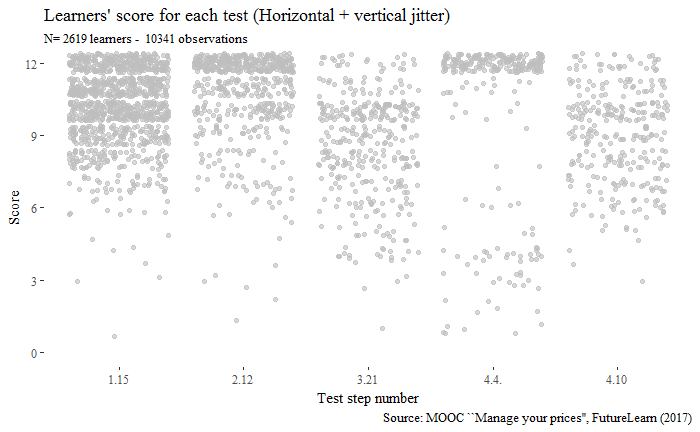### How cheating helps for ploting lines in parallel plots

Now, if we want to follow learners results over time (over tests), we can une parallel plots and draw lines linking each result.

``````#Spaghetti plot original
Plot.spaghetti <-  ggplot(Scorebylearners,
aes(x=step, y= test_score,
group=factor(learner_id))) +
guides(colour=FALSE) +
scale_x_discrete(name="Test step number", limits=c("1.15", "2.12", "3.21" , "4.4.", "4.10")) +
scale_y_discrete(name ="Score",  limits=c(0,3,6,9, 12)) +
labs(title = "Learners' score for each test  (Parallel plot)",
subtitle = paste("N=", nrow(Scorebylearners), "learners - ", nrow(TestAnalysis),"observations"),
caption = "Source: MOOC ``Manage your prices'', FutureLearn (2017)"
) +
coord_cartesian(ylim = c(0,12)) +
theme_tufte()

#Plot Spaghetti brut
Plot.spaghetti +
geom_line( color="grey", size=1) +
theme_tufte()``````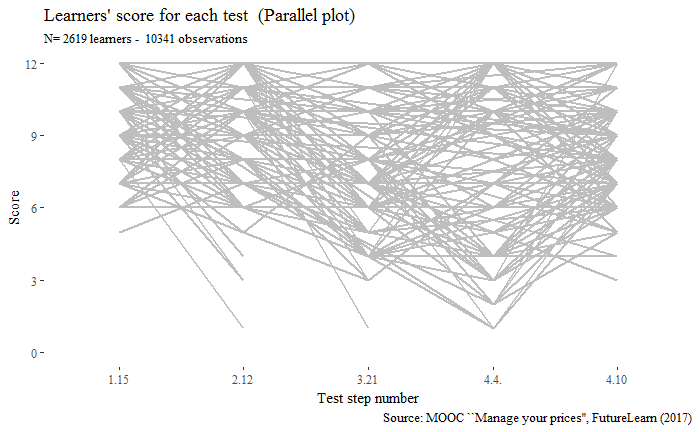Since the score range from 1 to 12 and are discrete. many lines overlap and it is quite impossible to see some “pattern” in learners score. Nothing emerges from this simultation.

Let us cheat a little bit

``````#Adding jitter on Ys, and alpha-brushing
Plot.spaghetti +
geom_line(alpha=0.30, color="grey", size=1,
aes(y = jitter(test_score, 2), x = step , group=factor(learner_id))) +
theme_tufte()``````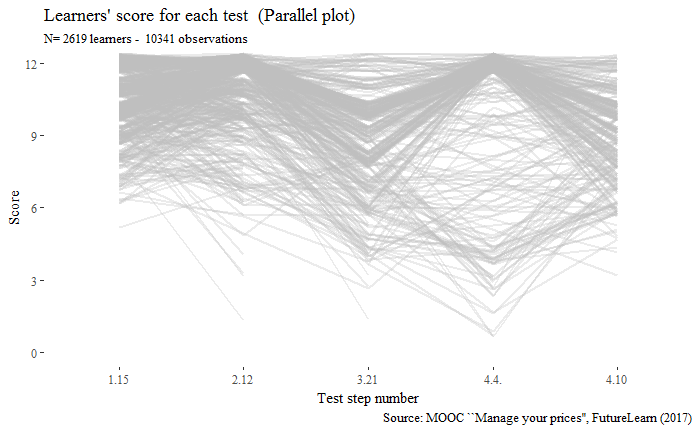Now we see it !

The difference between the two graph is quite striking. By adding some vertical noise on the Y axis - that is modifying randomly the score value so that it is not integer any more - and using some brushing , help revealing some unseen and unnoticed patterns.

``````#